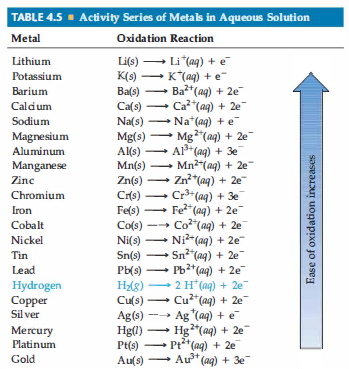# Problem: Based on the activity series (Table 4.5), what is the outcome (if any) of each of the following reactions?(a) Mn(s) + NiCl2(aq) →

###### Problem Details

Based on the activity series (Table 4.5), what is the outcome (if any) of each of the following reactions?
(a) Mn(s) + NiCl2(aq) →